• +91 9971497814
• info@interviewmaterial.com

# Chapter 9 Sequences and Series Ex-9.2 Interview Questions Answers

### Related Subjects

Question 1 : Find the sum of odd integers from 1 to 2001.

The odd integers from1 to 2001 are 1, 3, 5, …1999, 2001.

It clearly forms asequence in A.P.

Where, the firstterm, a = 1

Commondifference, d = 2

Now,

a + (n -1)d = 2001

1 + (n-1)(2) = 2001

2n – 2 = 2000

2n = 2000 + 2 = 2002

n = 1001

We know,

Sn =n/2 [2a + (n-1)d]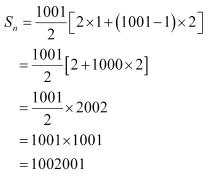Therefore, the sum ofodd numbers from 1 to 2001 is 1002001.

Question 2 : Find the sum of all natural numbers lying between 100 and 1000, which are multiples of 5.

The natural numbers lying between 100 and 1000, which are multiples of 5, are 105, 110, … 995.
It clearly forms a sequence in A.P.
Where, the first term, a = 105
Common difference, d = 5
Now,
a + (n -1)d = 995
105 + (n – 1)(5) = 995
105 + 5n – 5 = 995
5n = 995 – 105 + 5 = 895
n = 895/5
n = 179
We know,
Sn = n/2 [2a + (n-1)d]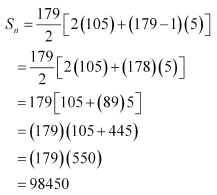Therefore, the sum of all natural numbers lying between 100 and 1000, which are multiples of 5, is 98450.

Question 3 : In an A.P, the first term is 2 and the sum of the first five terms is one-fourth of the next five terms. Show that 20th term is –112.

Given,

The first term (a) ofan A.P = 2

Let’s assume d bethe common difference of the A.P.

So, the A.P. will be2, 2 + d, 2 + 2d, 2 + 3d, …

Then,

Sum of first fiveterms = 10 + 10d

Sum of next five terms= 10 + 35d

From the question, wehave

10 + 10d = ¼ (10 +35d)

40 + 40d = 10 + 35d

30 = -5d

d = -6

a20 =a + (20 – 1)d = 2 + (19) (-6) = 2 – 114 = -112

Therefore, the 20th termof the A.P. is –112.

Question 4 : How many terms of the A.P. -6, -11/2, -5, …. are needed to give the sum –25?

Let’s consider the sum of n terms of the given A.P. as –25.
We known that,
Sn = n/2 [2a + (n-1)d]
where n = number of terms, a = first term, and d = common difference
So here, a = –6
d = -11/2 + 6 = (-11 + 12)/ 2 = 1/2
Thus, we have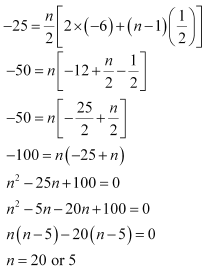Question 5 : In an A.P., if pth term is 1/q and qth term is 1/p, prove that the sum of first pq terms is ½ (pq + 1) where p ≠ q.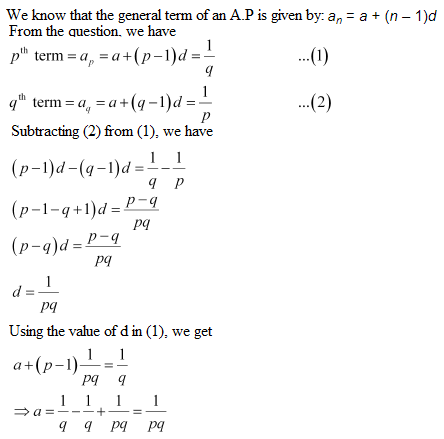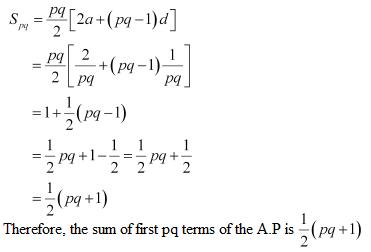Question 6 : If the sum of a certain number of terms of the A.P. 25, 22, 19, … is 116. Find the last term

Given A.P.,

25, 22, 19, …

Here,

First term, a = 25 and

Common difference, d =22 – 25 = -3

Also given, sum ofcertain number of terms of the A.P. is 116

The number of terms ben

So, we have

Sn =n/2 [2a + (n-1)d] = 116

116 = n/2 [2(25) +(n-1)(-3)]

116 x 2 = n [50 – 3n +3]

232 = n [53 – 3n]

232 = 53n – 3n2

3n2 –53n + 232 = 0

3n2 –24n – 29n+ 232 = 0

3n(n – 8) – 29(n – 8)= 0

(3n – 29) (n – 8) = 0

Hence,

n = 29/3 or n = 8

As n can only be anintegral value, n = 8

Thus, 8th termis the last term of the A.P.

a8 =25 + (8 – 1)(-3)

= 25 – 21

= 4

Question 7 : Find the sum to n terms of the A.P., whose kth term is 5k + 1.

Given, the kth termof the A.P. is 5k + 1.

kth term = ak = +(k – 1)d

And,

+ (k – 1)d = 5k +1

a + kd – d =5k + 1

On comparing thecoefficient of k, we get d = 5

– = 1

a – 5 = 1

a =6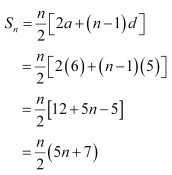Question 8 :

If the sum of n terms of an A.P. is (pn qn2),where p and q are constants, find the commondifference.

We know that,

Sn =n/2 [2a + (n-1)d]

From the question wehave,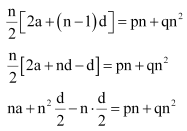On comparing thecoefficients of n2 on both sides, we get

d/2 = q

Hence, d =2q

Therefore, the commondifference of the A.P. is 2q.

Question 9 : The sums of n terms of two arithmetic progressions are in the ratio 5n + 4: 9n + 6. Find the ratio of their 18th terms.

Let a1a2,and d1dbe the firstterms and the common difference of the first and second arithmetic progressionrespectively.

Then, from thequestion we have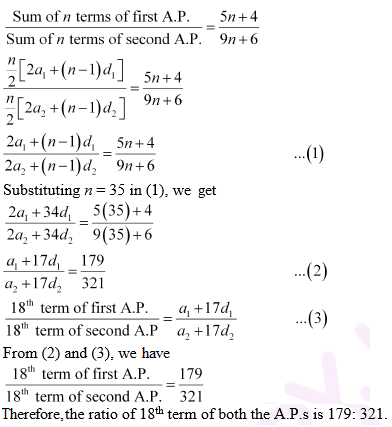Question 10 : If the sum of first p terms of an A.P. is equal to the sum of the first q terms, then find the sum of the first (p + q) terms.

Let’s take a and d tobe the first term and the common difference of the A.P. respectively.

Then, it given that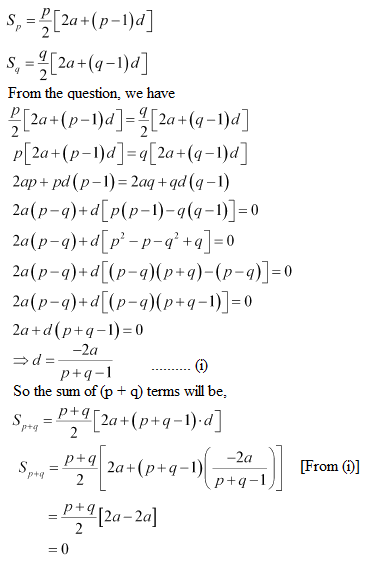Therefore, the sum of(p + q) terms of the A.P. is 0.

Todays Deals### Chapter 9 Sequences and Series Ex-9.2 Contributorskrishan

Name:
Email:

# Latest News# 9000 interview questions in different categories